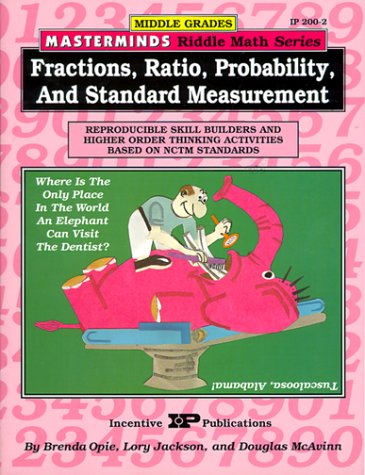# Masterminds Riddle Math for Middle Grades: Fractions, Ratio, Probability, and Standard Measurement: Reproducible Skill Builders and Higher Order Thinking Activities Based on NCTM Standards

• ISBN-13: 9780865303027
• \$10.58

Will be in stock after

Motivate students to find equivalent fractions; convert mixed numerals; multiply and divide all kinds of fractions; explore probability; work with standard measurements; simplify, multiply, and divide fractions; and use ratios, rates, and proportions to solve problems with the challenging math riddles in this book. All of the skills are based on NCTM standards and each page is an engaging and humorous puzzle.